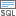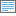# Extract by Attributes (Spatial Analyst)

## Summary

Extracts the cells of a raster based on a logical query.

## Usage

• Additional attributes from the input raster, if any, will be carried over as-is to the output raster attribute table. Depending on the property being recorded, some of the attribute values may need to be recalculated.

• When a multiband raster is specified as the Input Raster (in_raster in Python), all bands will be used.

To process a selection of bands from a multiband raster, first create a new raster dataset composed of those particular bands using the Composite Bands tool. Then use the result as the Input Raster (in_raster in Python).

The default output format is a geodatabase raster. If an Esri Grid stack is specified as the output format, the name of the stack cannot start with a number, use spaces, or be more than nine characters in length.

• If the Where clause evaluates to true, the original input value is returned for the cell location. If it evaluates to false, the cell location is assigned NoData.

• The Where clause uses an SQL query. See the following topics for more details on creating queries:

• In order to use a {where_clause} in Python, it should be enclosed in quotes. For example, "Value > 5000".

You can consult the help for more information on specifying a query in Python.

• If an item other than Value of input raster is specified in the query, the original input value is returned for the cell location.

• If the input raster is integer, the output raster will be integer. If the input is floating point, the output will be floating point.

• See Analysis environments and Spatial Analyst for additional details on the geoprocessing environments that apply to this tool.

## Parameters

 Label Explanation Data Type Input raster The input raster from which cells will be extracted. Raster Layer Where clause A logical expression that selects a subset of raster cells.The Where clause follows the general form of an SQL expression. It can be entered directly, for example, VALUE > 100, if you click the Edit SQL mode button. If in the Edit Clause Mode, you can begin constructing the expression by clicking on the Add Clause Mode button. SQL Expression

### Return Value

 Label Explanation Data Type Output raster The output raster containing the cell values extracted from the input raster. Raster

`ExtractByAttributes(in_raster, where_clause)`
 Name Explanation Data Type in_raster The input raster from which cells will be extracted. Raster Layer where_clause A logical expression that selects a subset of raster cells.The expression follows the general form of an SQL expression. An example of a where_clause is "VALUE > 100". SQL Expression

### Return Value

 Name Explanation Data Type out_raster The output raster containing the cell values extracted from the input raster. Raster

### Code sample

ExtractByAttributes example 1 (Python window)

This example extracts cells from a raster based on a logical query, where elevation is greater than 1,000 meters.

``````import arcpy
from arcpy import env
from arcpy.sa import *
env.workspace = "C:/sapyexamples/data"
attExtract = ExtractByAttributes("elevation", "VALUE > 1000")
attExtract.save("c:/sapyexamples/output/attextract")``````
ExtractByAttributes example 2 (stand-alone script)

This example extracts cells from a raster based on a logical query, where elevation is greater than 1,000 meters.

``````# Name: ExtractByAttributes_Ex_02.py
# Description: Extracts the cells of a raster based on a logical query.
# Requirements: Spatial Analyst Extension

# Import system modules
import arcpy
from arcpy import env
from arcpy.sa import *

# Set environment settings
env.workspace = "C:/sapyexamples/data"

# Set local variables
inRaster = "elevation"
inSQLClause = "VALUE > 1000"

# Execute ExtractByAttributes
attExtract = ExtractByAttributes(inRaster, inSQLClause)

# Save the output
attExtract.save("c:/sapyexamples/output/attextract02")``````

## Licensing information

• Basic: Requires Spatial Analyst
• Standard: Requires Spatial Analyst